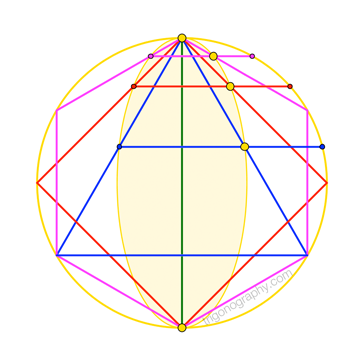Special Angles are GoldenThis is George Odom‘s elegant construction of the ever-fascinating golden ratio, $$\phi$$:

If $$a$$ measures a “midpoint segment” of an equilateral triangle, and if $$b$$ measures the extension of that segment meeting the triangle’s circumcircle, then $$\frac{a}{b} = \phi = 1.618\dots$$

As it turns out, Odom’s construction generalizes very nicely when we replace “midpoint segment” with “trisecting segment” or “quadrisecting segment”, and “equilateral triangle” with “square” or “regular hexagon”.
I became aware of the square result while contemplating this post to Math Stack Exchange by Dr. Elliot McGucken (aka, “Astrophysics Math”). This prompted my own (re?-)discovery of the hexagon result, which simply falls out of the straightforward analysis I provide below. It’s possible —even likely— that all of these observations already appear somewhere in the vast lore on the golden ratio, but I’m too lazy to do a literature search.
Update: The essence of the square construction appears in an isosceles-right-triangle construction
published in 2015 by Q. H. Tran (PDF). Curiously, despite noting the Odom-esque flavor of his result,
Tran doesn’t seem to realize that his figure features an obvious, and even-more-Odom-esque,
segment trisector. (The missed connection with the square is less surprising, given the semi-circular
context.) Nevertheless, it appears appropriate to assign priority to what I’ll call “Tran’s Square”.
Be that as it may …To see the underlying connections here, consider an inscribed angle of half-measure $$\theta$$, and suppose the “$$(n+1)$$-secting segment” through the sides of this angle is such that the ratio of its length to that of its circle-meeting extension is $$r : 1$$, for some not-necessarily-golden ratio $$r$$.
By the chord-chord aspect of the Power of a Point theorem, we have
$$n\cdot 1 = x \cdot ( x + r x )\quad\to\quad x = \sqrt{\frac{n}{1+r}}$$
But, clearly, we also have $$r x = 2\sin\theta$$, so that
$$\sin\theta = \frac{rx}{2} = \frac{\sqrt{n}}{2}\cdot\frac{r}{\sqrt{1+r}}\qquad(\star)$$
Now, recall that what makes the particular ratio $$\phi$$ “golden” is that the square of its value is precisely $$1$$ unit greater than the value itself; that is, $$\phi^2 = 1 + \phi$$. Consequently, when $$r = \phi$$, the second factor in $$(\star)$$ vanishes, leaving …

$$\sin\theta = \frac{\sqrt{n}}{2}$$
… which should be familiar to you
from the common mnemonic
involving trig’s “special angles” …
\begin{align} \sin \phantom{0}0^\circ &= \sqrt{0}\,/2 \\[4pt] \sin \color{blue}{30^\circ} &= \sqrt{\color{blue}{1}}\,/2 \quad\to\quad\text{Odom’s Triangle}\\[4pt] \sin \color{red}{45^\circ} &= \sqrt{\color{red}{2}}\,/2 \quad\to\quad\text{Tran’s Square}\\[4pt] \sin \color{violet}{60^\circ} &= \sqrt{\color{violet}{3}}\,/2 \quad\to\quad\text{Someone’s Hexagon} \\[4pt] \sin 90^\circ &= \sqrt{4}\,/2 \end{align}
In this very peculiar sense, then, special angles are indeed golden.Our sine relation establishes that $$n$$ cannot exceed four. Therefore, the triangle, square, and hexagon above represent all available (non-degenerate) Odom-like constructions for integer $$n$$. The composite view at right shows a bonus feature: All the dividing points lie on an ellipse. (No, the ellipse isn’t “golden”: neither its eccentricity, nor its aspect ratio, is $$\phi$$.)

Of course, no one requires $$n$$ to be an integer,
so there remains more to say. For instance, the Odom-like construction for the regular pentagon, which already admits so many connections with the golden ratio, has $$n = \phi^2 = \phi+1$$. Also, $$n = \phi$$ implies $$\theta \approx 39.49^\circ$$. (Is there anything (else) remarkable about that angle?) And so on.

I’ll leave further considerations to the reader.